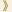HomeShaw Laureates2009Mathematical SciencesPress Release

Geometry and Physics have been closely related from the earliest times and the differential calculus of Newton and Leibniz became the common mathematical tool that connected them. The geometry of 2-dimensional surfaces was fully explored by these techniques in the 19th century. It was closely related to algebraic curves and also to the flow of fluids.

Extending our understanding to 3-dimensional space and 4-dimensional space-time has been fundamental for both geometers and physicists in the 20th and 21st centuries. While the calculus is still employed, the problems are now much deeper and totally new phenomena appear.

Simon K Donaldson and Clifford H Taubes are the two geometers who have transformed the whole subject by pioneering techniques and ideas originating in theoretical physics, including quantum theory.

Electromagnetism is governed by the famous differential equations of Clerk Maxwell and these equations were used in the early 20th century by William Hodge as geometric tools. They were particularly useful in the geometry associated with algebraic equations, extending the work of the 19th century mathematician Bernhard Riemann.

The physical forces involved in the atomic nucleus are governed by the Yang-Mills equations which generalize Maxwell’s equations but, being non-linear, are much deeper and more difficult. It was these equations which Donaldson used, basing himself on analytical foundations of Taubes, to derive spectacular new results. These opened up an entirely new field where more and more subtle geometric results have been established by Donaldson, Taubes and their students. The inspiration has frequently come from physics, but the methods are those of differential equations.

A key strand of this newly developing theory is the close relation that has been found between solutions of the Yang-Mills equations and the geometry of surfaces embedded in 4 dimensions. A definitive result in this direction is a beautiful theorem of Taubes which essentially identifies certain "quantum invariants" with others of a more classical nature. Many old conjectures have been settled by these new techniques, but many more questions still pose a challenge for the future. Donaldson and Taubes between them have totally changed our geometrical understanding of space and time.

Mathematical Sciences Selection Committee
The Shaw Prize

16 June 2009, Hong Kong